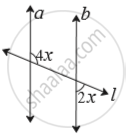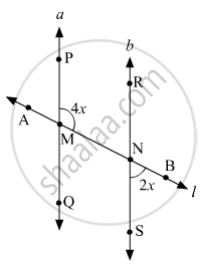SSC (English Medium) Class 8Maharashtra State Board
Share

# Choose the Correct Alternative. in the Adjoining Figure, If Line a Line B and Line L is a Transversal Then Find X. (A) 90° (B) 60° (C) 45° (D) 30° - SSC (English Medium) Class 8 - Mathematics

#### Question

Choose the correct alternative.

In the adjoining figure, if line a || line b and line l is a transversal then find x.• 90°

• 60°

• 45°

• 30°

#### SolutionLet us mark the points P and Q on a; R and S on b; A and B on l.
Suppose PQ and AB intersect at M; RS and AB intersect at N.
Since a||b and l is a transversal, then
m∠RNM = m∠SNB    (Vertically opposite angles)
⇒ ∠RNM  = 2x
Now, m∠RNM + m∠PMN  = 180°    (Interior angles on the same side of transversal are supplementary)
⇒ 2x + 4x = 180°
⇒ 6x = 180°

⇒ x = (180°)/6
⇒ x = 30°

So, the correct answer is option (D).

Is there an error in this question or solution?

#### APPEARS IN

Balbharati Solution for Balbharati Class 8 Mathematics (2019 to Current)
Chapter 2: Parallel lines and transversals
Practice Set 2.2 | Q: 1.2 | Page no. 8
Solution Choose the Correct Alternative. in the Adjoining Figure, If Line a Line B and Line L is a Transversal Then Find X. (A) 90° (B) 60° (C) 45° (D) 30° Concept: Transversal.
S